# 2021年成人高考高起点《理数》模拟试题七

2021-03-05 15:06 江西成考网

1.条件甲：“|z-1|≤1”是条件乙：“|z|≤2”的（   ）

A.必要非充分条件
B.充分非必要条件
C.充分必要条件
D.既非必要也非充分条件

2.A.36
B.220
C.660
D.1320

3.

4..下列等式中，成立的是（   ）A.A
B.B
C.C
D.D

5.下列函数中奇函数的个数为 （    ）A.1个
B.2个
C.3个
D.4个

5.（    ）

A.5
B.-5
C.5/2
D.-5/2

6.（    ）

A.-1 ≤ x ＜-1/2
B.-1/2 ＜ x ≤ 1
C. 0 ≤ x＜ 2π/3
D.2π/3 ＜ x ≤ π

7.（    ）

A.P=-1,q=6
B.P=1,q=6
C.P=-1,q=-6
D.P=1,q=-6

8.设a是第四象限角，则以下函数值一定是负值的是（   ）

A.cos 2a
B.C.D.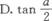9.(x-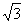)10的展开式中，x6的系数握（    ）

A.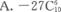B.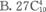C.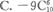D.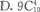10.A.A
B.B
C.C
D.D

11.下列函数中，为偶函数且在区间(0，+∞)上单调递减的函数是 （　　）

A.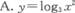B.C.D.12.A.- lg(x+1)
B.- lg(1-x)
C.lg(1-x)
D.lg(x+1)2 /2

13A.A
B.B
C.C
D.D

14.抛物线顶点在坐标原点，焦点在y轴上，其上的点P（m，-3）到焦点的距离为5，则抛物线的方程为（   ）

A.x2=4y
B.x2=-4y
C.x2=-8y
D.x2=8y

15.A.A
B.B
C.C
D.D

16.直线2x+3y-6=0关于y轴对称的直线方程是（   ）

A.2x-3y-6=0
B.2x-3y+6=0
C.2x+3y+6=0
D.2x+3y-6=0

17.设函数y=ax+2的反函数为它的自身，则a的值为（　　）

A.±1
B.1
C.-1
D.4

18.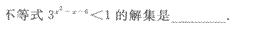19.在(1+a)+(1+a)2+…+(1+a)9的展开式中，各项系数之和是__________．

20.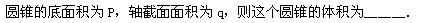21.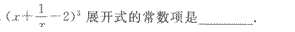22.23.24.如下图所示，把AB=3，BC=4的矩形ABCD沿对角线AC折成120。的二面角，求点B、D的距离．

25.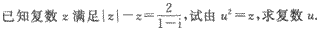437
2021年成人高考考试时间
10月23,24日+